# Hausdorff implies sober

Jump to: navigation, search
This article gives the statement and possibly, proof, of an implication relation between two topological space properties. That is, it states that every topological space satisfying the first topological space property (i.e., Hausdorff space) must also satisfy the second topological space property (i.e., sober space)
View all topological space property implications | View all topological space property non-implications
Get more facts about Hausdorff space|Get more facts about sober space

## Statement

Any Hausdorff space is a sober space.

## Definitions used

### Hausdorff space

Further information: Hausdorff space

A topological space$X$ is said to be Hausdorff if, for any two points$x,y$ in$X$, there exist open subsets$U,V$ of$X$ such that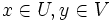$x \in U, y \in V$ and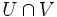$U \cap V$ is empty.

### Sober space

Further information: sober space

A topological space$X$ is termed sober if the only irreducible closed subsets of$X$ are the closures of one-point subsets.

## Proof

We execute this proof by showing that, for a Hausdorff space, the only irreducible closed subsets are the one-point subsets. Note that this in particular shows that the only irreducible closed subsets are the closures of one-point subsets.

Given: A topological space$X$, an irreducible closed subset$A$ of$X$.

To prove:$A$ is a one-point subset.

Proof: Since$A$ is irreducible, it is nonempty, so it has at least one point. We show that if$A$ has two points, we obtain a contradiction.

Suppose$x,y \in A$ are two distinct points. By the definition of Hausdorffness, there exist open subsets$U,V$ of$X$ such that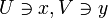$U \ni x, V \ni y$, and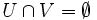$U \cap V = \emptyset$. Then, the sets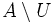$A \setminus U$ and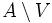$A \setminus V$ are both closed subsets, their union is$A$, and they are both proper subsets of$A$ since$x \notin A \setminus U$ and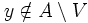$y \notin A \setminus V$. Thus, we have written$A$ as a union of two proper closed subsets, contradicting the assumption of irreducibility.